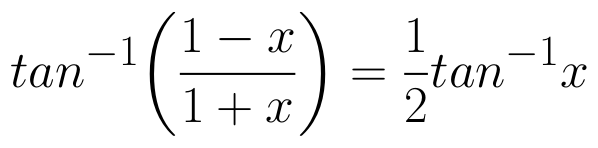Select Page

# MCQ in English Answers for CBSE Maths 12 Science Differential Equations

MCQ in English Answers for CBSE Maths 12 Science Differential Equations to enable students to get Answers in a narrative video format for the specific question.

Expert Teacher provides MCQ Answers for CBSE Maths 12 Science Differential Equations through Video Answers in English language. This video solution will be useful for students to understand how to write an answer in exam in order to score more marks. This teacher uses a narrative style for a question from Differential Equations not only to explain the proper method of answering question, but deriving right answer too.

Please find the question below and view the Answer in a narrative video format.

Question:

## Similar Questions from CBSE, 12th Science, Maths, Differential Equations

Question 1 : Find the particular solution of the differential equation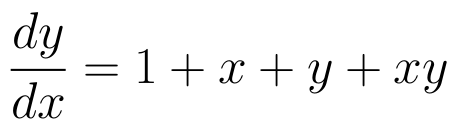given that y = 0 when x = 1. (View Answer Video)

Question 2 : Find the particular solution of the differential equation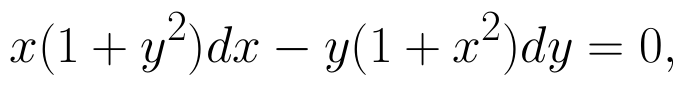given that y = 1, when x = 0. (View Answer Video)

Question 3 : Form the differential equation of equation y = a cos 2x + b sin 2x, where a and b are constant. (View Answer Video)

Question 4 : Solve the differential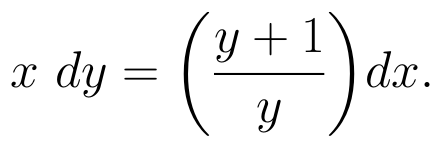(View Answer Video)

Question 5 : Form the differential equation of the family of circles in the second quadrant and touching the co-ordinate axes. (View Answer Video)

### Relations and Functions

Question 1 : The identity element for the binary operation * defined by a * b =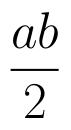, where a, b are the elements of a set of non-zero rational numbers, is, (View Answer Video)

Question 2 : A function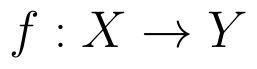is bijective if and only if, (View Answer Video)

Question 3 : Letbe defined as f(x) = 3x. Choose the correct answer. (View Answer Video)

Question 4 : Let * be the binary operation on N given by a * b = LCM of a and b. Find 20 * 16. (View Answer Video)

Question 5 : Let * be the binary operation on N given by a * b = LCM of a and b. Find the identity of * in N. (View Answer Video)

### Application of Integrals

Question 1 : Using the method of integration, find the area of the region bounded by the lines 3x - y - 3 = 0, 2x + y - 12 = 0 and x -2y - 1 = 0. (View Answer Video)

Question 2 : Using integration find the area of region bounded by the triangle whose vertices are (-1, 0), (1, 3) and (3, 2). (View Answer Video)

Question 3 : Given that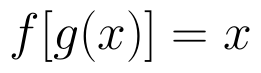, for x = 0 to x = 20. Find f(x) and g(x) such that the area between f(x) and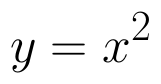from x = 0 to x = 5 be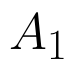and area between g(x) and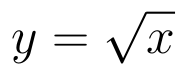from y = 0 to y = 5 be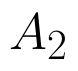. Is? Like functions f and g which work is better, team work or individual work? (View Answer Video)

Question 4 : Find the area of the smaller part of the circle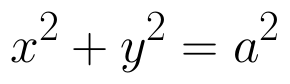cut off by the line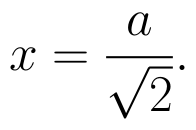(View Answer Video)

Question 5 : Find the area enclosed between the parabola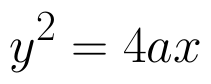and the line y = mx. (View Answer Video)

### Inverse Trigonometric Functions

Question 1 : Write the principal value of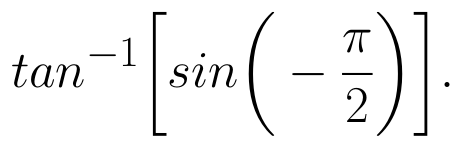(View Answer Video)

Question 2 : Write in the simplest form: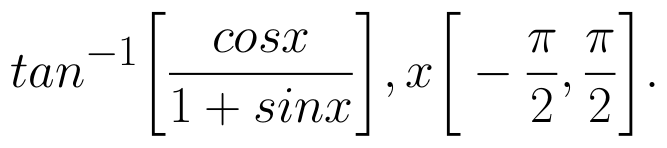(View Answer Video)

Question 3 : Write the value of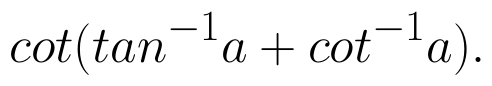(View Answer Video)

Question 4 : Write the principal value of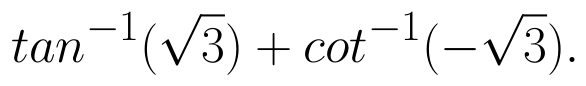(View Answer Video)

Question 5 :

Solve the equation: